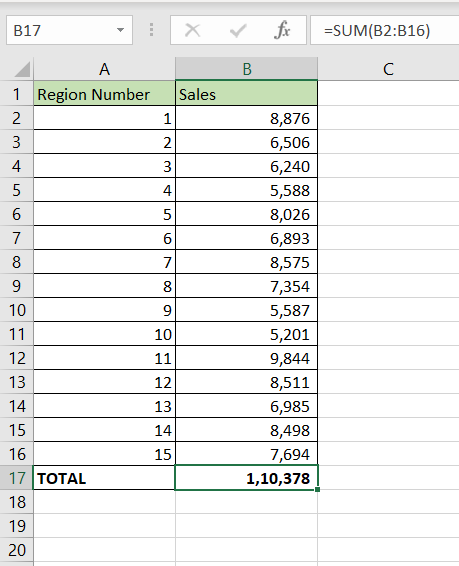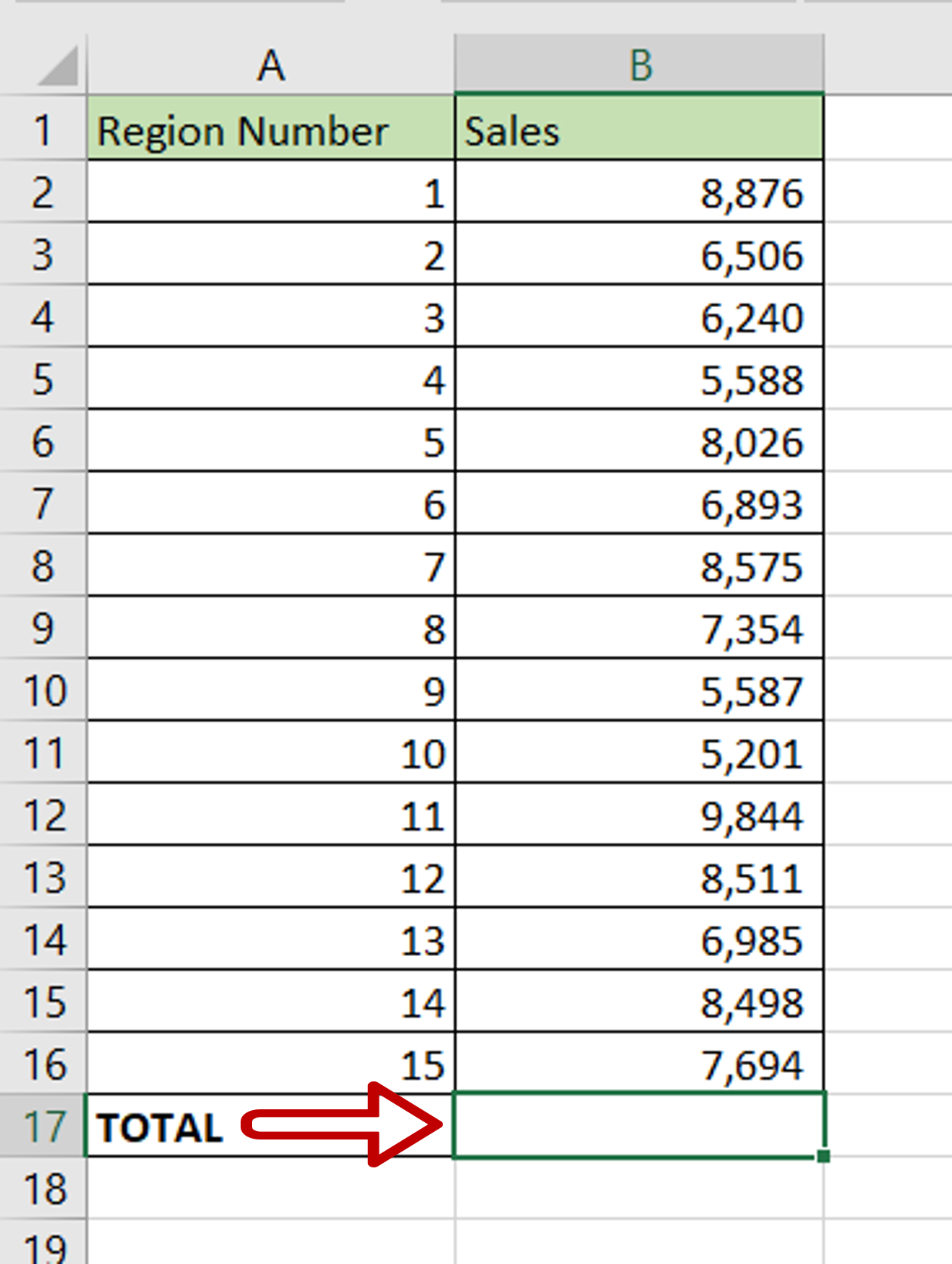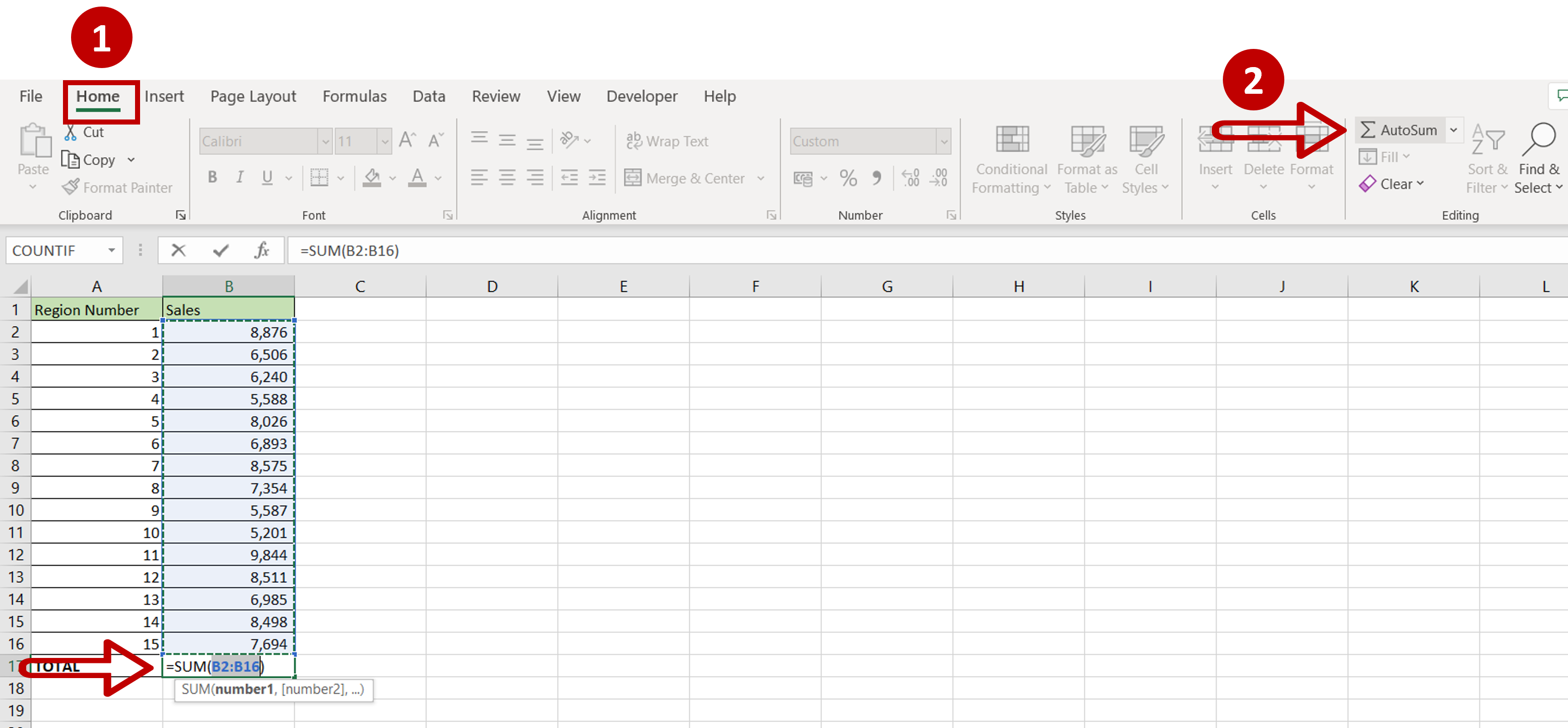# How to autosum a column in Excel

You can watch a video tutorial here.Excel is widely used for calculations due to the several arithmetic operators and functions that it has. Instead of typing in numbers for calculations, you can use the cell references i.e. the address (Column header & Row number) of the cell in the calculation. When adding a range of cells in Excel, you can do so using the cell references of the start and end of the range. Excel has a useful feature called AutoSum that can be used to sum numbers in a column.

### Step 1 – Select the cell– Select the cell at the end of the column in which the result is to appear

### Step 2 – Use AutoSum– Go to Home > Editing
– Click the AutoSum button
– The SUM() function with the range of cells will be automatically populated in the cell
– Press Enter

Note: AutoSum will automatically populate the range only if the cell for the result is at the end of the column. If the cell for the result is elsewhere on the sheet, you will need to select the range of cells before using AutoSum

### Step 3 – Check the result– The sum of the column is displayed